# Image Compression with Wavelets¶

Important: Please read the installation page for details about how to install the toolboxes. $\newcommand{\dotp}{\langle #1, #2 \rangle}$ $\newcommand{\enscond}{\lbrace #1, #2 \rbrace}$ $\newcommand{\pd}{ \frac{ \partial #1}{\partial #2} }$ $\newcommand{\umin}{\underset{#1}{\min}\;}$ $\newcommand{\umax}{\underset{#1}{\max}\;}$ $\newcommand{\umin}{\underset{#1}{\min}\;}$ $\newcommand{\uargmin}{\underset{#1}{argmin}\;}$ $\newcommand{\norm}{\|#1\|}$ $\newcommand{\abs}{\left|#1\right|}$ $\newcommand{\choice}{ \left\{ \begin{array}{l} #1 \end{array} \right. }$ $\newcommand{\pa}{\left(#1\right)}$ $\newcommand{\diag}{{diag}\left( #1 \right)}$ $\newcommand{\qandq}{\quad\text{and}\quad}$ $\newcommand{\qwhereq}{\quad\text{where}\quad}$ $\newcommand{\qifq}{ \quad \text{if} \quad }$ $\newcommand{\qarrq}{ \quad \Longrightarrow \quad }$ $\newcommand{\ZZ}{\mathbb{Z}}$ $\newcommand{\CC}{\mathbb{C}}$ $\newcommand{\RR}{\mathbb{R}}$ $\newcommand{\EE}{\mathbb{E}}$ $\newcommand{\Zz}{\mathcal{Z}}$ $\newcommand{\Ww}{\mathcal{W}}$ $\newcommand{\Vv}{\mathcal{V}}$ $\newcommand{\Nn}{\mathcal{N}}$ $\newcommand{\NN}{\mathcal{N}}$ $\newcommand{\Hh}{\mathcal{H}}$ $\newcommand{\Bb}{\mathcal{B}}$ $\newcommand{\Ee}{\mathcal{E}}$ $\newcommand{\Cc}{\mathcal{C}}$ $\newcommand{\Gg}{\mathcal{G}}$ $\newcommand{\Ss}{\mathcal{S}}$ $\newcommand{\Pp}{\mathcal{P}}$ $\newcommand{\Ff}{\mathcal{F}}$ $\newcommand{\Xx}{\mathcal{X}}$ $\newcommand{\Mm}{\mathcal{M}}$ $\newcommand{\Ii}{\mathcal{I}}$ $\newcommand{\Dd}{\mathcal{D}}$ $\newcommand{\Ll}{\mathcal{L}}$ $\newcommand{\Tt}{\mathcal{T}}$ $\newcommand{\si}{\sigma}$ $\newcommand{\al}{\alpha}$ $\newcommand{\la}{\lambda}$ $\newcommand{\ga}{\gamma}$ $\newcommand{\Ga}{\Gamma}$ $\newcommand{\La}{\Lambda}$ $\newcommand{\si}{\sigma}$ $\newcommand{\Si}{\Sigma}$ $\newcommand{\be}{\beta}$ $\newcommand{\de}{\delta}$ $\newcommand{\De}{\Delta}$ $\newcommand{\phi}{\varphi}$ $\newcommand{\th}{\theta}$ $\newcommand{\om}{\omega}$ $\newcommand{\Om}{\Omega}$

This numerical tour uses wavelets to perform image compression. We consider a simple model for compression, where we only estimate the number of bits of the compressed data, without really performing the actual entropic coding.

In :
addpath('toolbox_signal')

[Warning: Function isrow has the same name as a MATLAB builtin. We suggest you
rename the function to avoid a potential name conflict.]
[> In path at 110
In pymat_eval at 38
In matlabserver at 27]
[Warning: Function isrow has the same name as a MATLAB builtin. We suggest you
rename the function to avoid a potential name conflict.]
[> In path at 110
In pymat_eval at 38
In matlabserver at 27]


## Wavelet Domain Quantization¶

Image compression is perfomed by first quantizing the wavelet coefficients of an image.

A scalar quantizer of step size |T| uses the function |floor|. It has a twice larger zero bins.

Create values evenly spaced for quantization.

In :
v = linspace(-1,1, 2048);


Bin size for the quantization. The larger, the more agressive the compression.

In :
T = .1;


For compression, we compute quantized integer values.

In :
vI = floor(abs(v/T)).*sign(v);


For decompression, we compute de-quantized values from |vI|, which are chosen as the mid-point of each quantization bin.

In :
vQ = sign(vI) .* (abs(vI)+.5) * T;


Display the quantization curve.

In :
clf;
subplot(1,2,1);
plot(v, vI);
axis('tight');
title(strcat(['Quantized integer values, T=' num2str(T)]));
subplot(1,2,2);
hold('on');
plot(v, vQ);
plot(v, v, 'r--');
axis('equal'); axis('tight');
title('De-quantized real values');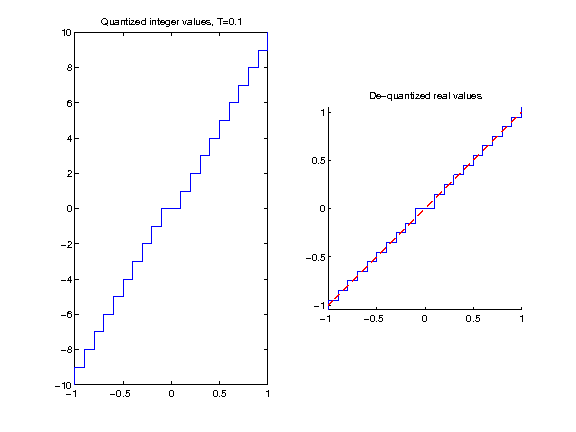## Quantization and Approximation of Wavelet Coefficients¶

Quantization of wavelet coefficients set to 0 those coefficients which are smaller than |T|, but it also modify the values of larger coeffiients. It thus creates an error that is slightly larger than simply performing an approximation with hard thresholding at |T|.

In :
n = 256;
M = rescale( load_image('lena', n) );


Compute its wavelet transform.

In :
Jmin = 4;
MW = perform_wavelet_transf(M,Jmin, +1);


Exercise 1

Compute the coefficients |MWT| obtained by thresholding at |T| the coefficients |MW|. Compute the coefficients |MWQ| obtained by quantizing with bin size |T| the same coefficients. Display them using the function |plot_wavelet|. hresholding approximation isplay

In :
exo1()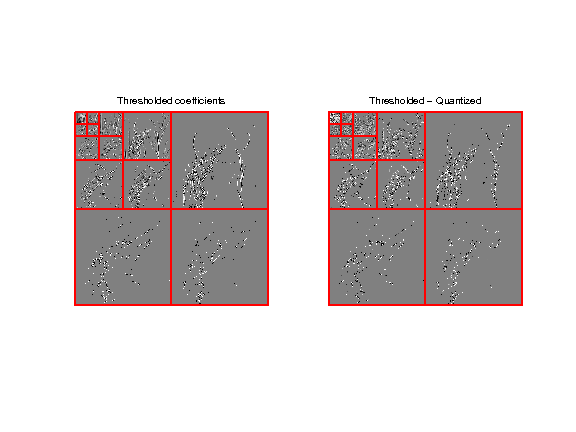In :
%% Insert your code here.


Exercise 2

Compare the effect of quantizing at |T=.2| and thresholding at |T=.2| the wavelet coefficients of an image. nverse transform rror isplay

In :
exo2()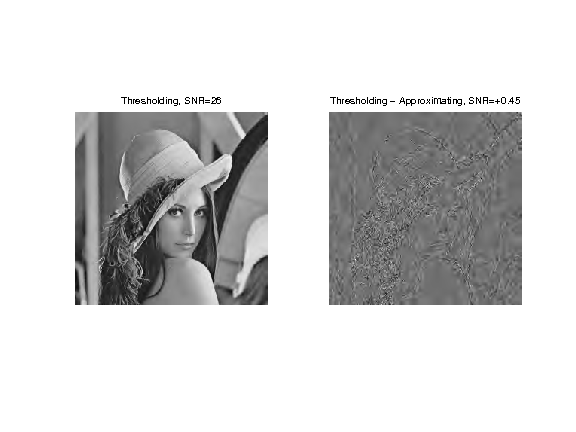In :
%% Insert your code here.


Exercise 3

Compute a bin size |T0| to quantize the original |M| itself to obtained |MQ0| such that |norm(M-MQ,'fro')| is as close as possible to the error obtained with wavelet domain quantization.

In :
exo3()

Spatial quantization step T0=0.089.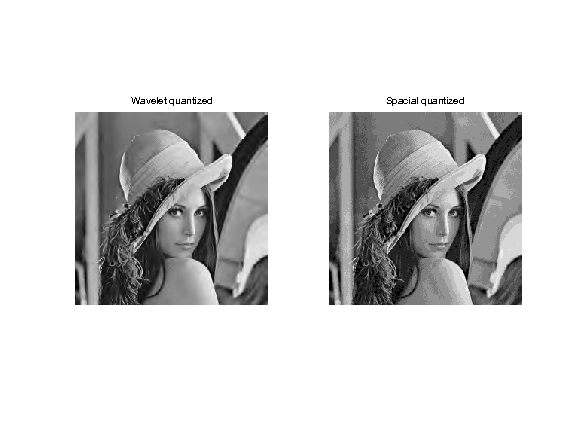In :
%% Insert your code here.


## Entropy Coding the Wavelet Coefficients¶

Actually store the quantized coefficients in a file, one need to compute a binary code from |MWI|. The length of this code is the number of bits used by the compressor, which typically increases when |T| decays toward 0.

To reduce the number of bits, an entropic coder makes use of the statistical distribution of the quantized values.

First we quantize the coefficients.

In :
MWI = floor(abs(MW/T)).*sign(MW);
MWQ = sign(MWI) .* (abs(MWI)+.5) * T;


Assuming that all the coefficients of |MWI| are drawn independently from the same distribution with histogram |h|, the minium bit per pixel achievable is the Entropy lower bound.

|E = -\sum_i \log2(h(i))*h(i)|

Huffman trees and more precisely block-Huffman tree codes get increasingly closer to this bound when the data size increases. Arithmetic coders also achieves very good results and are fast to compute.

Compute the nomalized histogram of the quantized wavelet coefficients.

In :
a = max(abs(MWI(:)));
t = -a:a;
h = hist(MWI(:), t); h = h/sum(h);


Compute the histogram of the quantized pixels or the original image.

In :
t0 = 0:1/T0;
MI = floor(abs(M/T0)); % quantized pixel values
h0 = hist(MI(:), t0); h0 = h0/sum(h0);


Display the histograms.

In :
clf;
subplot(2,1,1);
bar(t0,h0); axis('tight');
title('Pixels');
subplot(2,1,2);
bar(t,h); axis([-5 5 0 max(h)])
title('Wavelets (zoom)');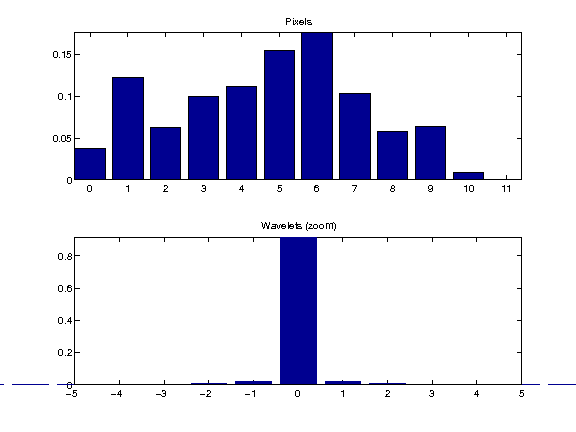Exercise 4

Compute the entropy lower bound for the quantized wavelet coefficients and for the quantized pixel values. Take care of |log2(0)| when |h(i)=0|.

In :
exo4()

Pixels entropy:  3.2
Wavelet entropy: 0.72

In :
%% Insert your code here.


Exercise 5

Compute, for various threshold |T|, the number of bits per pixels |E(T)| of the quantized wavelet coefficients, and the wavelet decompression error |err(T)|, compute using SNR. Display the rate distortion curve |err| as a function of |E|.

In :
exo5()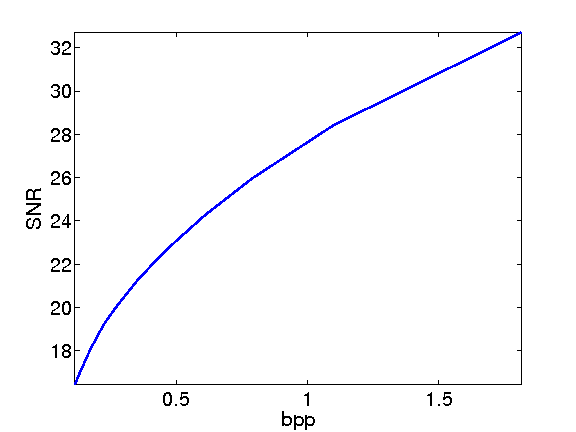In :
%% Insert your code here.


## Scale-by-scale Entropy Coding¶

Wavelet coefficients of an image does not have the same distribution accross the scales. Taking this into account can further reduce the number of bits for coding.

Quantize the coeffients.

In :
T = .1;
MWI = floor(abs(MW/T)).*sign(MW);


Extact the fine scale wavelet coefficients.

In :
MWH = MWI(1:n/2,n/2+1:n);
MWV = MWI(n/2+1:n,1:n/2);
MWD = MWI(n/2+1:n,n/2+1:n);


Display.

In :
clf;
imageplot(MWH,'Horizontal',1,3,1);
imageplot(MWV,'Vertical',1,3,2);
imageplot(MWD,'Diagonal',1,3,3);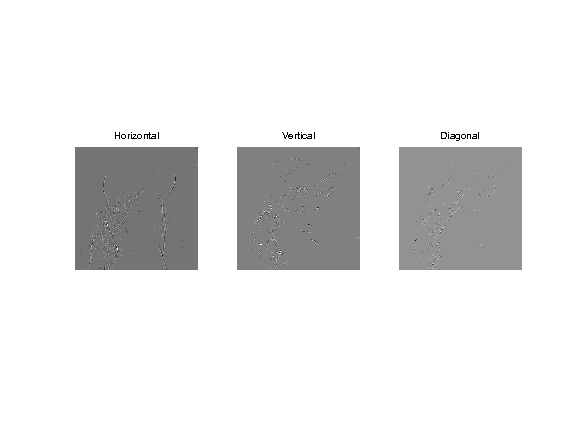Exercise 6

Extract the three fine scale wavelet coefficients (horizontal, vertical, diagonal directions) and quantize them, for instance with |T=.1|. Compute the entropy of the three sets together, and compute the entropy of each set.

In :
exo6()

Entropy, all:  0.287
Entropy, hor:  0.466
Entropy, vert: 0.212
Entropy, diag: 0.157

In :
%% Insert your code here.


Exercise 7

Compare the number of bits needed to code all the wavelet coefficients together, and the number of bits needed to code independantly each scale of wavele coefficients for |Jmin=4<=j<=log2(n)-1| (and group together the remaining coefficients for |j<Jmin|).

In :
exo7()

nb.bis, whole:    0.719 bpp
nb.bis, separate: 0.58 bpp

In :
%% Insert your code here.


Exercise 8

Compute the rate distortion curve obtained by coding the coefficient separately through the scale, and compare with the rate distortion curve obtained by coding the coefficients as a whole.

In :
exo8()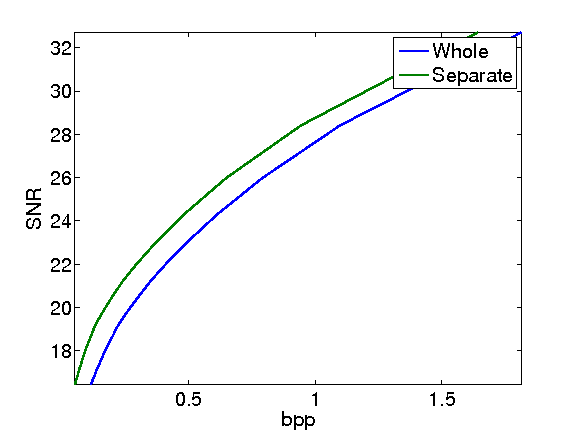In :
%% Insert your code here.